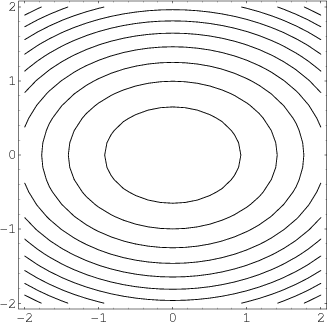# Math Insight

### Image: Elliptic paraboloid level curvesLevel curves of the elliptic paraboloid $f(x,y)=-x^2-2y^2=c$ for $c=-1,-2, \ldots, -10$. These curves are ellipses of increasing size.

Image file: elliptic_paraboloid_level_curves.png